☎  +49 9135 73666 65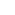0# Switching power supply operation

## 1.Step: rectification of the mains voltage

A 1-, 2- or 3-phase mains voltage (AC) is applied to the input of an AC/DC power supply. For convenience, the operation is explained using a 1-phase 230V (50Hz) voltage as an example. The diode is the decisive electronic component for rectification. It is only permeable in one direction, the other direction is blocked. Like a check valve.
Since with only one diode, as in picture left, only a half-wave is produced and thus much is "given away", one optimizes this by the rectifier method two-pulse rectifier (picture right).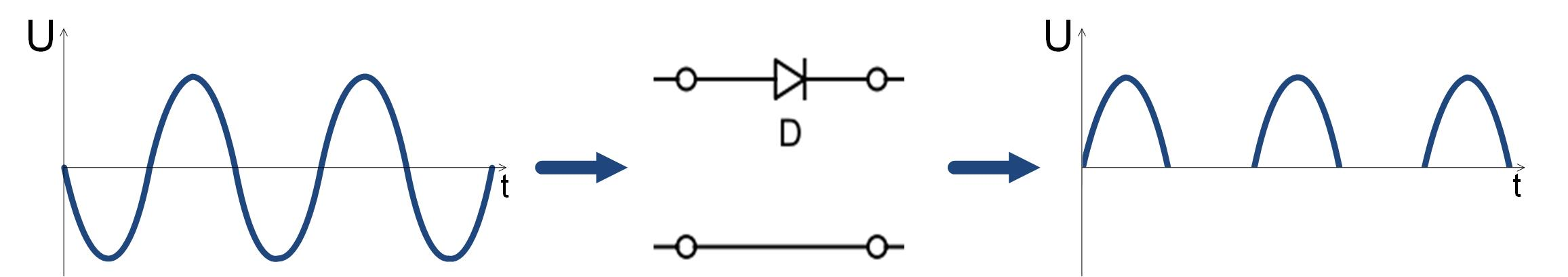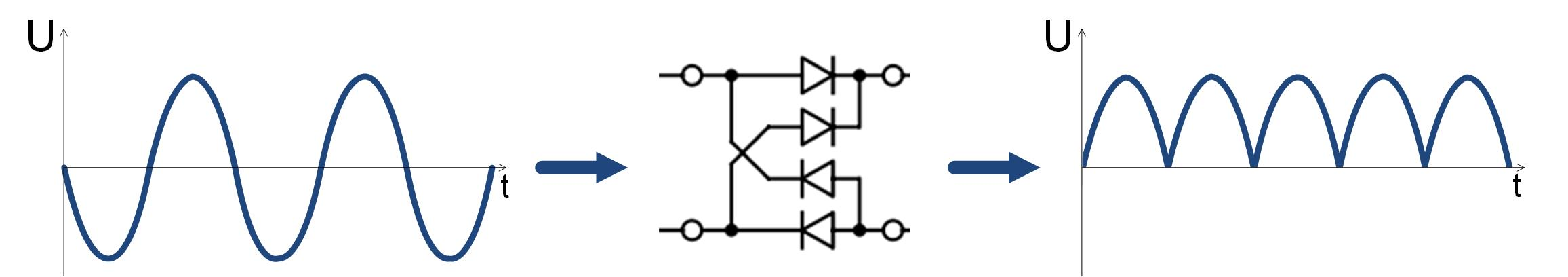## 2.Step: voltage smoothing by line filter

However, the voltage is now not yet permanently present; it also drops to 0V. Constantly switching it on and off would put a heavy load on a connected electronic circuit.
. To fill these "voltage holes", or buffer, a passive 2nd order low pass is used in this example. (Figure 2) The capacitor C stores the voltage, or is charged, and then releases it when our supply voltage decreases. The storage inductor keeps the current flow nearly constant. How far the voltage then drops varies by the size of the capacitor and the current flowing.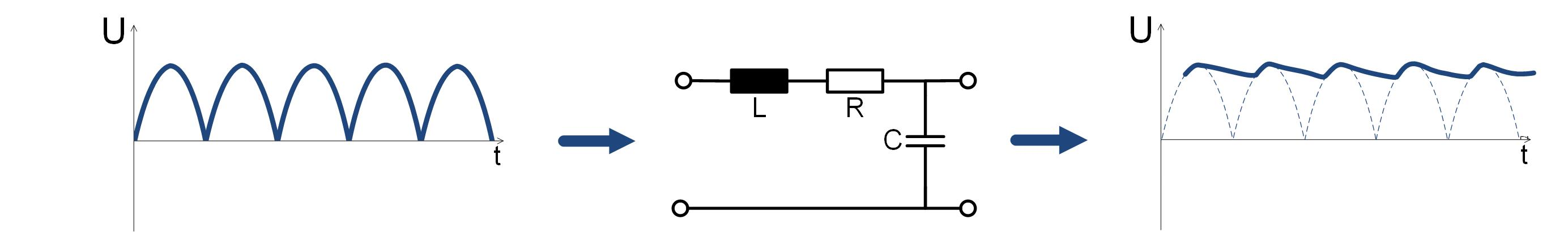## 3.Step: Convert DC input voltage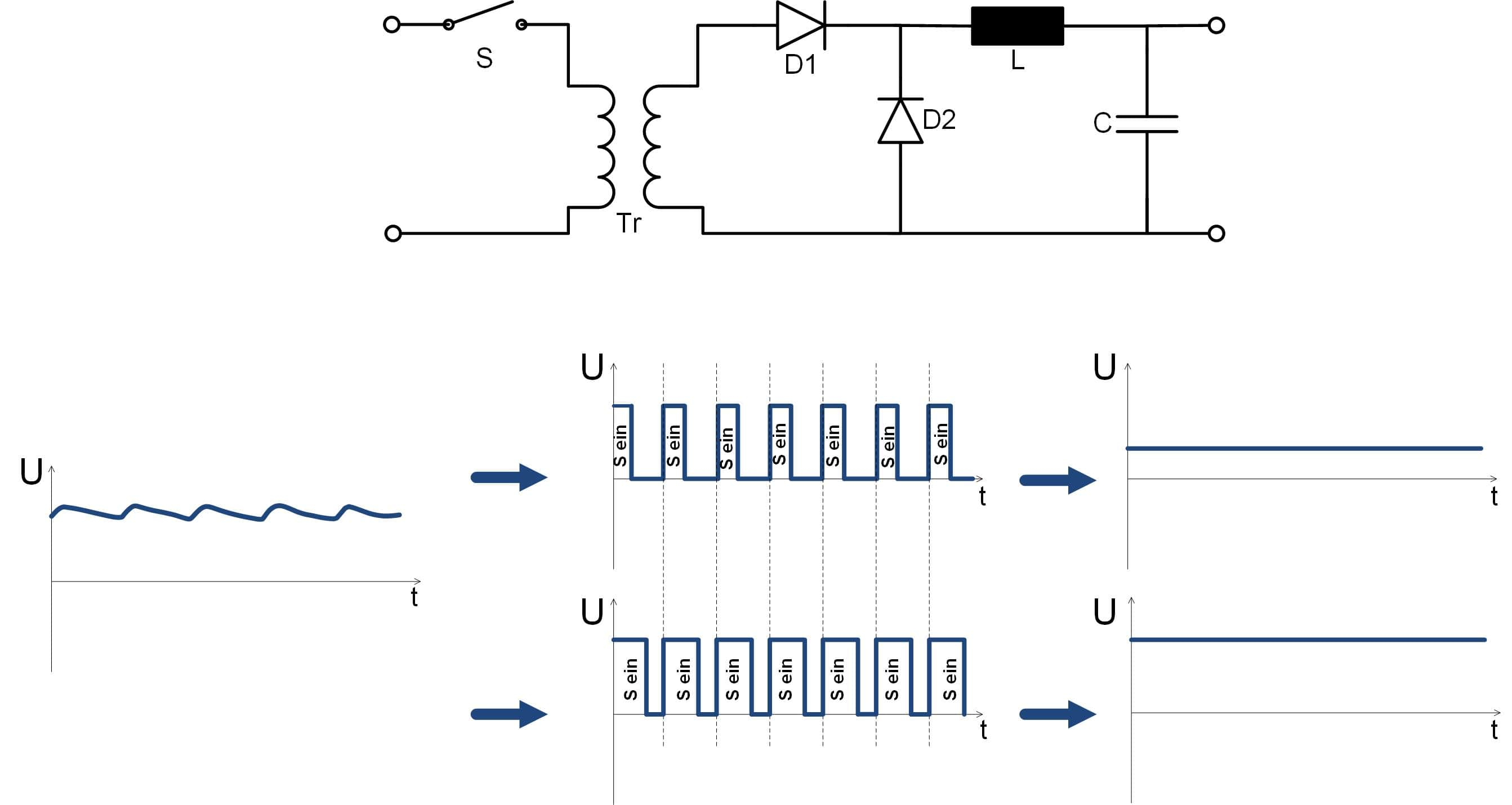Now the rectified, smoothed line voltage (about 325V) still has to be converted to the desired output voltage value. Thus a DC/DC conversion takes place. In this example (Fig. 3), the circuit topology of the single-ended forward converter is selected. However, other topologies are possible.
The switch S corresponds to a switching transistor, which is controlled with switching frequencies of some 10 kHz up to some 100 kHz and load-dependent pulse widths by a control unit not shown.

The transformer Tr represents the galvanic isolation and converts the input voltage to a secondary-side voltage according to the number of turns ratio.
In the conducting state, when the switch S is closed, a current flows through the primary winding of the transformer Tr and a current translated by the turns number ratio flows through the output-side diode D1 and the storage choke L. The diode D2 blocks in this state. The current increases linearly due to the buildup of a magnetic field in the storage choke. The capacitor C is charged to the output voltage.
The energy flow takes place in the conducting phase.

In the blocking state, the switch S is open and D1 blocks as the secondary voltage reverses polarity. The storage choke L allows a steady current to flow through the then conducting D2 during the blocking phase. Together with the capacitor C, the output voltage UA is thus kept constant, except for a small ripple.
The output voltage is load dependent and must be controlled by feedback and control of the switching transistors, not shown in Fig. 3.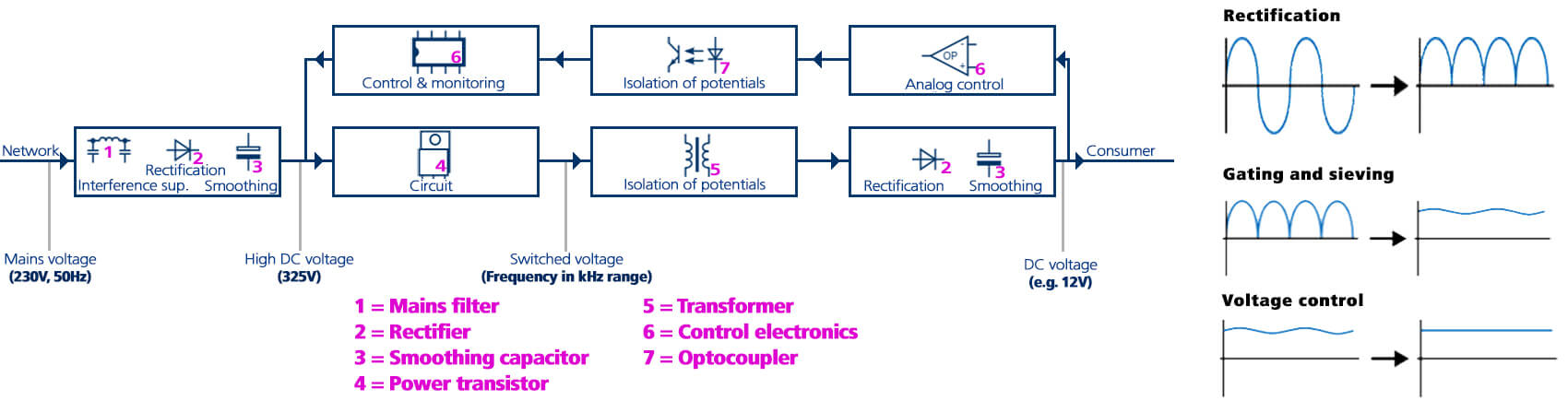The interaction of the individual steps of the voltage conversion is once again summarized in the block diagram (Figure 4). This diagram also includes the control unit feedback.